## 介绍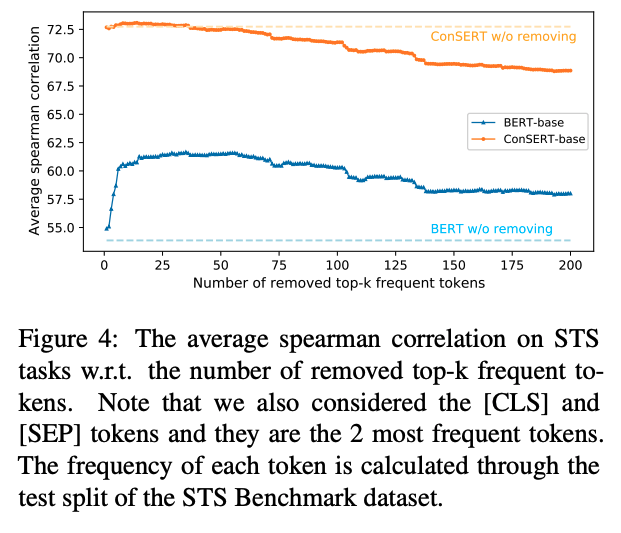## 方法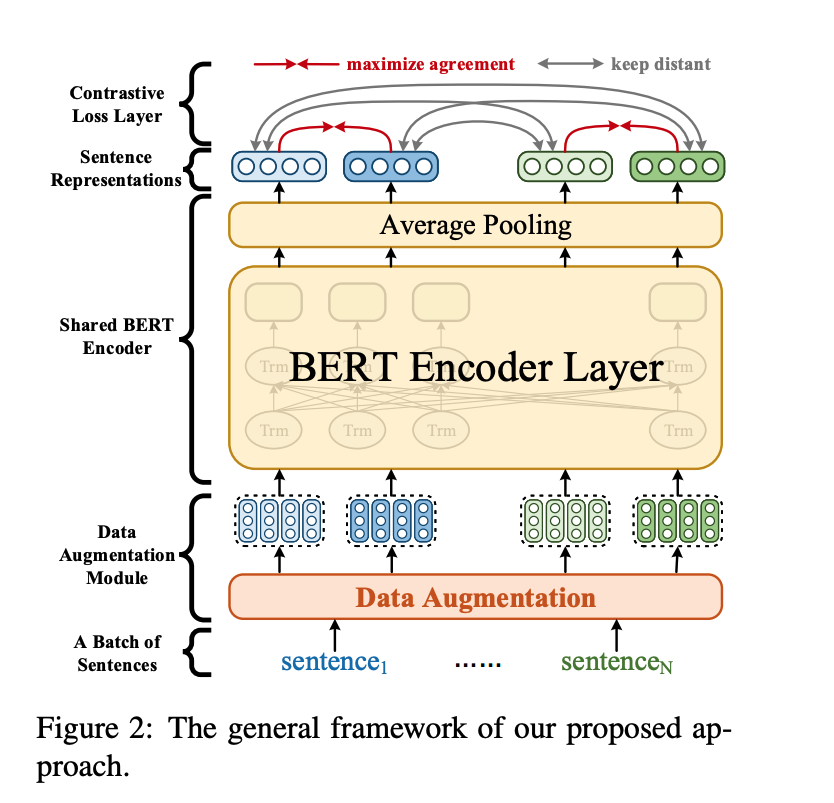• 数据增强模块: 作用于Embedding层，为同一个句子生成两个不同的增强版本（View）。
• 共享的BERT编码器，为输入的句子生成句向量。
• 对比损失层: 用于在一个Batch的样本中计算对比损失，其思想是最大化同一个样本不同增强版本句向量的相似度，同时使得不同样本的句向量相互远离。

$\mathcal{L}_{i, j}=-\log \frac{\exp \left(\operatorname{sim}\left(r_{i}, r_{j}\right) / \tau\right)}{\sum_{k=1}^{2 N} \mathbb{1}_{[k \neq i]} \exp \left(\operatorname{sim}\left(r_{i}, r_{k}\right) / \tau\right)}$

### 数据增强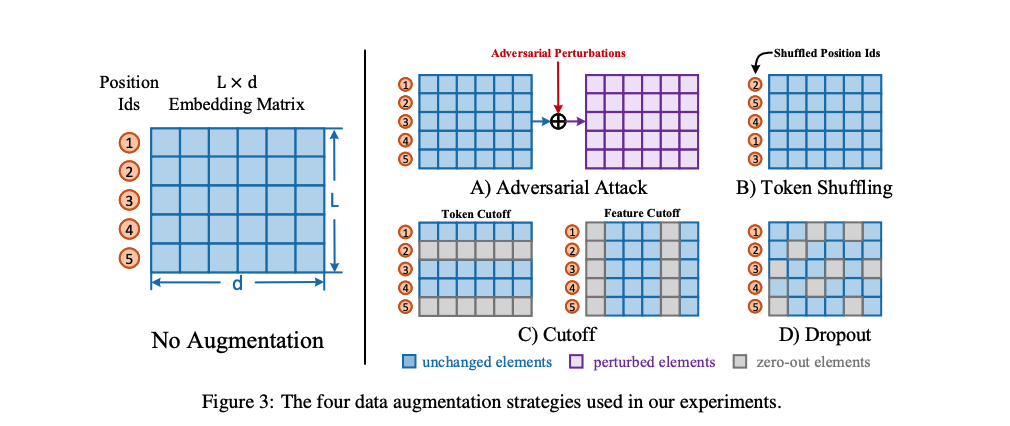• 打乱词序（Token Shuffling）：这一方法扰乱输入样本的词序。由于Transformer结构没有“位置”的概念，模型对Token位置的感知全靠Embedding中的Position Ids得到。因此在实现上，只需要将Position Ids进行Shuffle即可。

• Token Cutoff：随机选取Token，将对应Token的Embedding整行置Dropout。

• feature Cutoff：随机选取feature 维度，将对应Embedding整列置Dropout。

• dropout：Embedding中的每一个元素都以一定概率置为零。

### 训练策略

• 联合训练（joint）：有监督的损失和无监督的损失通过加权联合训练模型，即：$L_{joint} = L_{ce} + \alpha L_{con}$$L_{con}$表示对比损失，$L_{ce}$表示CrossEntropy损失。

• 先有监督再无监督（sup-unsup）：先使用有监督损失训练模型，再使用无监督的方法进行表示迁移。

• 联合训练再无监督（joint-unsup）：先使用联合损失训练模型，再使用无监督的方法进行表示迁移。

## 实验

### 实验数据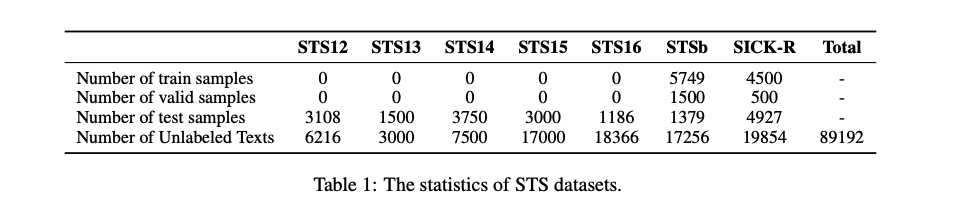### 实验结果

1. 无监督实验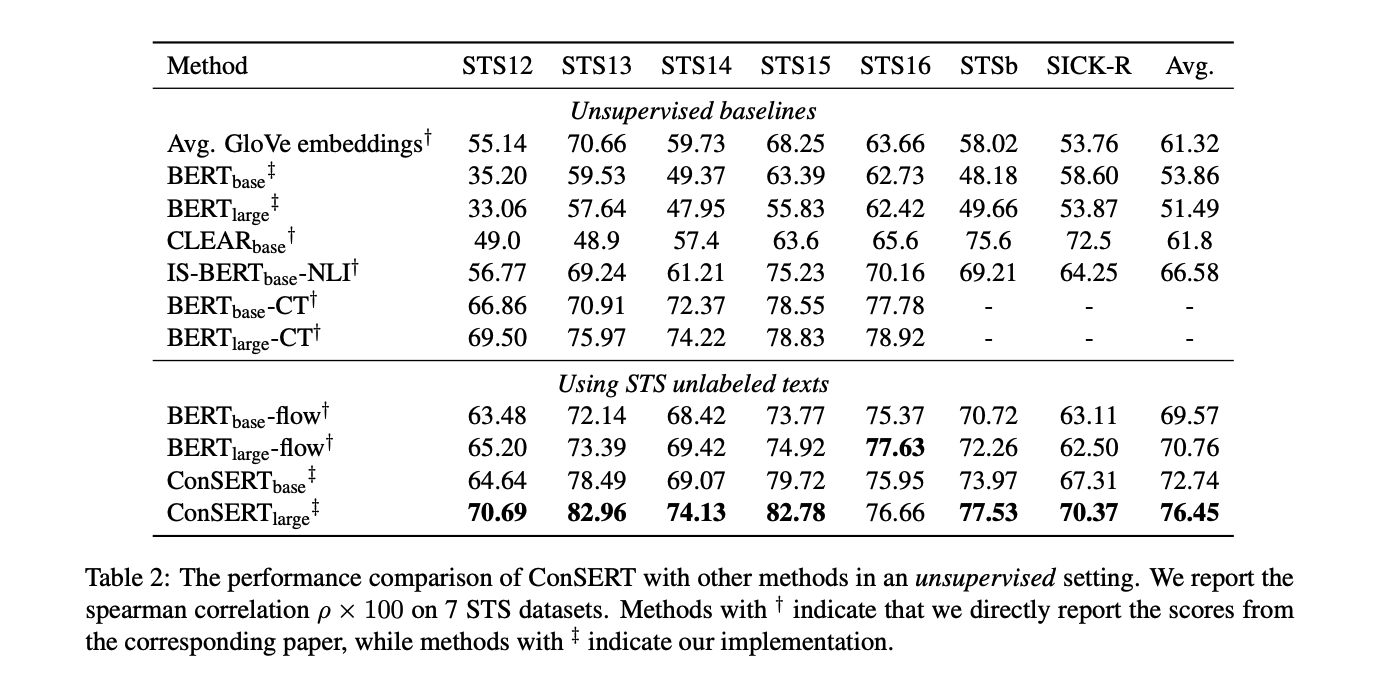1. 有监督实验1. 少样本实验1. 不同数据增强方法实验1. $\tau$超参数实验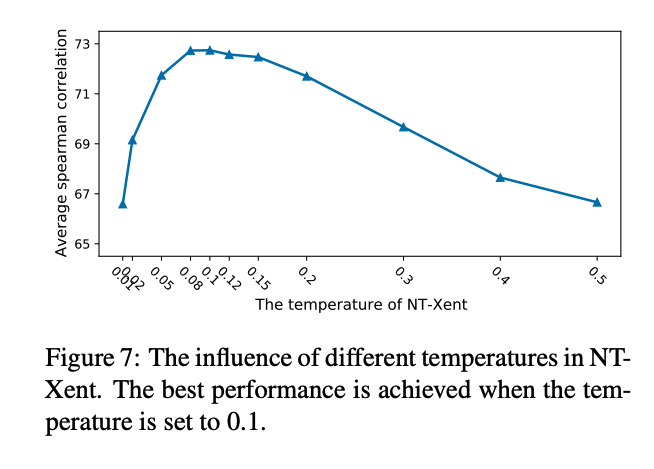$\tau$超参数实验中，从上图可以看出，对比学习损失函数中的温度超参数$\tau$对于结果有很大影响。当值在0.08到0.12之间时会得到最优结果。这个现象再次证明了BERT表示的Collapse问题，因为在句子表示都很接近的情况下，过大会使句子间相似度更平滑，编码器很难学到知识。而如果过小，任务就太过简单，所以需要调整到一个合适的范围内。